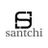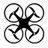## Combining UAV-based hyperspectral imagery and machine learning algorithms for soil moisture content monitoring609 days ago
RT @DroneNewsFeed: Combining UAV-based hyperspectral imagery and machine learning algorithms for soil moisture conten... https://t.co/r2aqS…RT @DroneNewsFeed: Combining UAV-based hyperspectral imagery and machine learning algorithms for soil moisture conten... https://t.co/r2aqS…Combining UAV-based hyperspectral imagery and machine learning algorithms for soil moisture conten... https://t.co/r2aqSU3jQp #drone #uav
NOT PEER-REVIEWED
"PeerJ Preprints" is a venue for early communication or feedback before peer review. Data may be preliminary.

A peer-reviewed article of this Preprint also exists.

View peer-reviewed version

## Supplemental Information

### r2 maps of 2D spectral indices based on R

(A) r2 maps of R_DI(479,619). (B) r2 maps of R_RI(431,446). (C) r2 maps of R_NDI(431,446). (D) r2 maps of R_PI(446,471). The colorbar illustrates the value of the square of the correlation coefficient (r2) between SMC and spectral indices, and the x- axes and y-axes indicate the wavebands of 400–1000 nm. Dark red portrays a high r2 between SMC and the spectral indices.

### r2 maps of 2D spectral indices based on FDR

(A) r2 maps of FDR_DI(435,746). (B) r2 maps of FDR_RI(702,724). (C) r2 maps of FDR_NDI(702,726). (D) r2 maps of FDR_PI(435,744). The colorbar illustrates the value of the square of the correlation coefficient (r2) between SMC and spectral indices, and the x- axes and y-axes indicate the wavebands of 400–1000 nm. Dark red portrays a high r2 between SMC and the spectral indices.

### r2 maps of 2D spectral indices based on SDR

(A) r2 maps of SDR_DI(710,753). (B) r2 maps of SDR_RI(444,895). (C) r2 maps of SDR_NDI(417,753). (D) r2 maps of SDR_PI(653,753). The colorbar illustrates the value of the square of the correlation coefficient (r2) between SMC and spectral indices, and the x- axes and y-axes indicate the wavebands of 400–1000 nm. Dark red portrays a high r2 between SMC and the spectral indices.

### r2 maps of 2D spectral indices based on CR

(A) r2 maps of CR_DI(400,446). (B) r2 maps of CR_RI(431,446). (C) r2 maps of CR_NDI(431,446). (D) r2 maps of CR_PI(446,466). The colorbar illustrates the value of the square of the correlation coefficient (r2) between SMC and spectral indices, and the x- axes and y-axes indicate the wavebands of 400–1000 nm. Dark red portrays a high r2 between SMC and the spectral indices.

### r2 maps of 2D spectral indices based on A

(A) r2 maps of A_DI(431,446) . (B) r2 maps of A_RI(431,619) . (C) r2 maps of A_NDI(431,619). (D) r2 maps of A_PI(446,471). The colorbar illustrates the value of the square of the correlation coefficient (r2) between SMC and spectral indices, and the x- axes and y-axes indicate the wavebands of 400–1000 nm. Dark red portrays a high r2 between SMC and the spectral indices.

### r2 maps of 2D spectral indices based on FDA

(A) r2 maps of FDA_DI(435,744) . (B) r2 maps of FDA_RI(420,726) . (C) r2 maps of FDA_NDI(513,726). (D) r2 maps of FDA_PI(435,713). The colorbar illustrates the value of the square of the correlation coefficient (r2) between SMC and spectral indices, and the x- axes and y-axes indicate the wavebands of 400–1000 nm. Dark red portrays a high r2 between SMC and the spectral indices.

### r2 maps of 2D spectral indices based on SDA

(A) r2 maps of SDA_DI(579,753) . (B) r2 maps of SDA_RI(440,446) . (C) r2 maps of SDA_NDI(477,753). (D) r2 maps of SDA_PI(753,946). The colorbar illustrates the value of the square of the correlation coefficient (r2) between SMC and spectral indices, and the x- axes and y-axes indicate the wavebands of 400–1000 nm. Dark red portrays a high r2 between SMC and the spectral indices.

### ELM algorithm

ELM algorithm code in matlab

### RF algorithm

RF algorithm code in matlab

### Spectral information extracted from UAV hyperspectral imagery

Reflectance of samples (n= 70)

### Competing Interests

The authors declare that they have no competing interests.

### Author Contributions

Xiangyu Ge conceived and designed the experiments, performed the experiments, analyzed the data, prepared figures and/or tables, approved the final draft.

Jingzhe Wang conceived and designed the experiments, analyzed the data, authored or reviewed drafts of the paper.

Jianli Ding authored or reviewed drafts of the paper, approved the final draft.

Xiaoyi Cao performed the experiments.

Zipeng Zhang performed the experiments.

Jie Liu contributed reagents/materials/analysis tools.

Xiaohang Li contributed reagents/materials/analysis tools.

### Data Deposition

The following information was supplied regarding data availability:

The RF and ELM algorithm are provided in the supplementary files Dataset S1 and Dataset S2.

The raw spectral data are provided in the supplementary files Dataset S3.

### Funding

This work was supported by the National Natural Science Foundation of China (41771470), and Program for Key Laboratory of Xinjiang Province, China (2016D03001). The funders had no role in study design, data collection and analysis, decision to publish, or preparation of the manuscript.

Some Markdown syntax is allowed: _italic_ **bold** ^superscript^ ~subscript~ %%blockquote%% [link text](link URL)